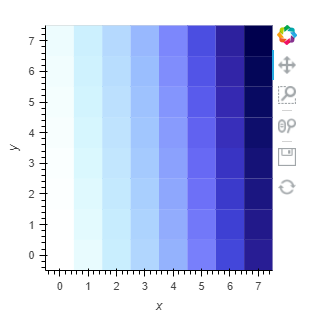# Supplying alpha values for Quadmesh

Based on my post from last year I’m still struggling with supplying alpha values to a HeatMap like object in a correct way. I found that I can pass dimension names, which worked very well at first.
However, I noticed that my data is often irregularly spaced, which is why I can’t use the HeatMap object for each case. For these cases I wanted to switch to QuadMesh which got me what I wanted for the general visualization (no white blocks between unevenly space values), however it doesn’t seem to support alpha dimension mapping yet. Below a short example.

``````import numpy as np
import holoviews as hv
from holoviews import opts
hv.extension('bokeh')

n = 8
xs = np.logspace(1, 3, n)
ys = np.linspace(1, 10, n)
zs = np.arange((n-1)**2).reshape(n-1, n-1)
alpha = zs / 48  # just an example

hv.QuadMesh((xs, ys, zs, alpha), kdims=["x", "y"], vdims=["z", "alpha"]).opts(alpha="alpha")
``````

This gives me the following error:

``````ValueError [Call holoviews.ipython.show_traceback() for details]
Mapping a dimension to the "alpha" style option is not supported by the QuadMesh element using the bokeh backend. To map the "alpha" dimension to the alpha use a groupby operation to overlay your data along the dimension.
``````

I didn’t get the groupby operation that is mentioned in the error to work for me here. How can I apply the correct alpha values for each point with QuadMesh?

Is there really no way I can supply individual alpha values to Quadmesh just like Heatmap supports it via an additional dimensions?

Here’s a truly inefficient way (unless you bin it better)

``````import numpy as np
import holoviews as hv
from holoviews import opts
hv.extension('bokeh')

n = 8
xs = np.logspace(1, 3, n)
ys = np.linspace(1, 10, n)
zs = np.arange((n)**2).reshape(n, n)
alpha = zs / 48  # just an example

import xarray as xr
ds = xr.merge([
xr.DataArray(zs, dims=("x", "y"), name="z"),
xr.DataArray(alpha, dims=("x", "y"), name="alpha")
])

# hv.QuadMesh((xs, ys, zs, alpha), kdims=["x", "y"], vdims=["z", "alpha"])

ds["alpha"] /= ds["alpha"].max()

hv.Overlay([hv.QuadMesh(ds.where(ds["alpha"] == alpha), kdims=["x", "y"], vdims=["z", "alpha"]).opts(alpha=alpha) for alpha in np.unique(ds["alpha"])])
``````